# RD Sharma Solutions (Ex.1.4): Rational Numbers Notes | Study RD Sharma Solutions for Class 8 Mathematics - Class 8

## Class 8: RD Sharma Solutions (Ex.1.4): Rational Numbers Notes | Study RD Sharma Solutions for Class 8 Mathematics - Class 8

The document RD Sharma Solutions (Ex.1.4): Rational Numbers Notes | Study RD Sharma Solutions for Class 8 Mathematics - Class 8 is a part of the Class 8 Course RD Sharma Solutions for Class 8 Mathematics.
All you need of Class 8 at this link: Class 8

Question 1: Simplify each of the following and write as a rational number of the form p/q: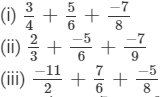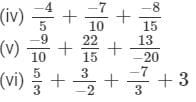(i)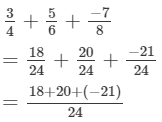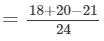=17/24

(ii)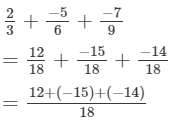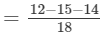= -17/18

(iii)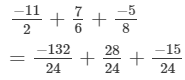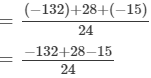= -119/24

(iv)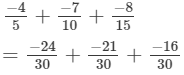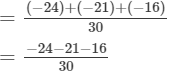= -61/31

(v)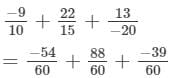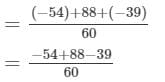= -5/60

= -1/12

(vi)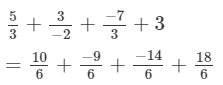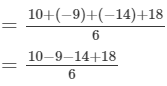= 5/6

Question 2: Express each of the following as a rational number of the form p/q: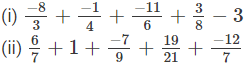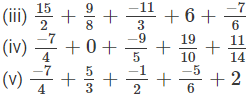(i)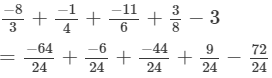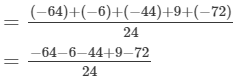= -177/24

= -59/8

(ii)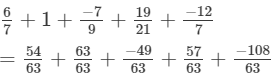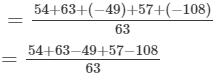= 17/63

(iii)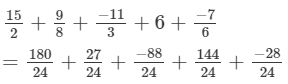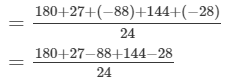= 235/24

(iv)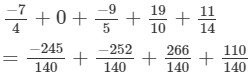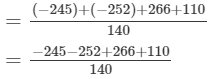= -121/140

(v)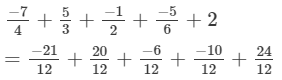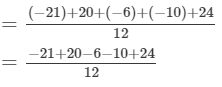= 7/12

Question 3: Simplify: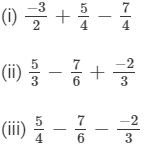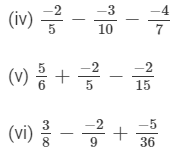(i)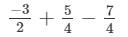Taking the L.C.M.of the denominators: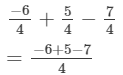-8/4

= -2

(ii)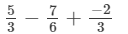Taking the L.C.M.of the denominators: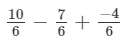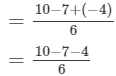= -1/6

(iii)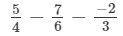Taking the L.C.M.of the denominators: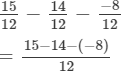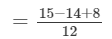= 9/12

= 3/4

(iv)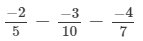Taking the L.C.M.of the denominators: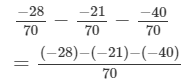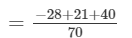= 33/70

(v)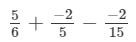Taking the L.C.M.of the denominators: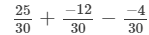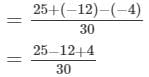= 17/30

(vi)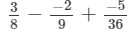Taking the L.C.M.of the denominators: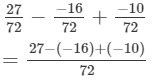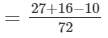= 33/72

= 11/24

The document RD Sharma Solutions (Ex.1.4): Rational Numbers Notes | Study RD Sharma Solutions for Class 8 Mathematics - Class 8 is a part of the Class 8 Course RD Sharma Solutions for Class 8 Mathematics.
All you need of Class 8 at this link: Class 8Use Code STAYHOME200 and get INR 200 additional OFF

## RD Sharma Solutions for Class 8 Mathematics

88 docs

Track your progress, build streaks, highlight & save important lessons and more!

,

,

,

,

,

,

,

,

,

,

,

,

,

,

,

,

,

,

,

,

,

;